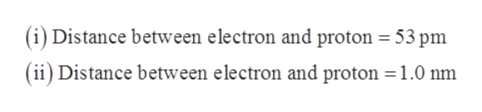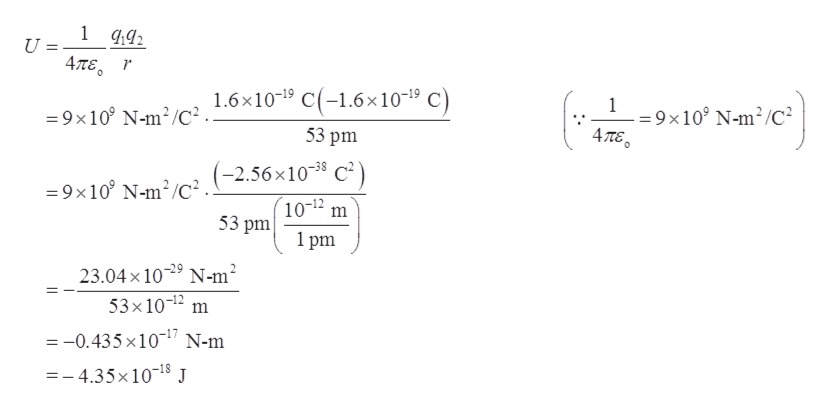# What is the electrostatic potential energy (in joules) between an electron and a proton that are separated by 53 pm? What is the Change in potential energy if the distance separating the electron and proton is increased to 1.0 nm? ) Does the potential energy of the two particles increase or decrease when the distance is increased to 1.0 nm?

Question
74 views

What is the electrostatic potential energy (in joules) between an electron and a proton that are separated by 53 pm? What is the Change in potential energy if the distance separating the electron and proton is increased to 1.0 nm? ) Does the potential energy of the two particles increase or decrease when the distance is increased to 1.0 nm?

check_circle

Step 1

The electrostatic potential energy between two charge is calculated by the coulomb’s law. The formula is written below.

Step 2

Given data:help_outlineImage Transcriptionclose(i) Distance between electron and proton = 53 pm (ii) Distance between electron and proton =1.0 nm fullscreen
Step 3

The charge on electron (q1) is 1.6 × 10-19 C and charge on proton (q2) is -1.6 × 10-19 C. Substitute the given value in equation (I) t...help_outlineImage Transcriptionclose1 492 4πε, . ( 1.6×10-19 C(-1.6×10-1º C) = 9x10° N-m?/C² . - = 9×10° N-m²/C² Απε, 53 pm -2.56x10-38 C²) = 9x10° N-m/C². 10-12 m 53 pm 1 pm 23.04 x 10-29 N-m² 53x10-12 m = -0.435 x10-" N-m =- 4.35×10-18 J fullscreen

### Want to see the full answer?

See Solution

#### Want to see this answer and more?

Solutions are written by subject experts who are available 24/7. Questions are typically answered within 1 hour.*

See Solution
*Response times may vary by subject and question.
Tagged in

### Physical Chemistry